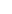It is widely used, combining in the best possible way the WMA's "hypersensitivity" and SMA’s "slowness". This is a weighted average of a price data, which adds more weight to the most recent data. Weighting the most recent value depends on each time period of the Moving Average. The shorter the EMA time period, the more weight is given to the most recent value.

We use the current closing value (not a rule) and the EMA value of the previous day (or any other time period we define) for the calculation. Specifically: EMA = (C-EMApr.) * K + EMApr., where

EMA= Exponential Moving Average.

C = current closing price (not a rule).

EMApr. = The average value of the previous time interval.

K = 2 / (n + 1), where n is the time period of interest.

As n increases, K decreases, and as a result EMA becomes less "sensitive" to price changes and approaches SMA.

When we first calculate EMA, we use the SMA as EMApr.

The main advantage of EMA compared to SMA is that it places more weight on the most recent observations and thus allows for a faster response to recent price changes. Also, unlike SMA and WMA, all previous data values ​​are used in the EMA calculation. Although the impact of past data is diminishing over time, it is never eliminated. The latter has as a result that even if the price remains unchanged for n intervals, SMA and EMA differ in price.to signal pageExponential Moving Average (EMA) ❺❺❺ - average rating 5 from 5 (based on 194 user reviews)